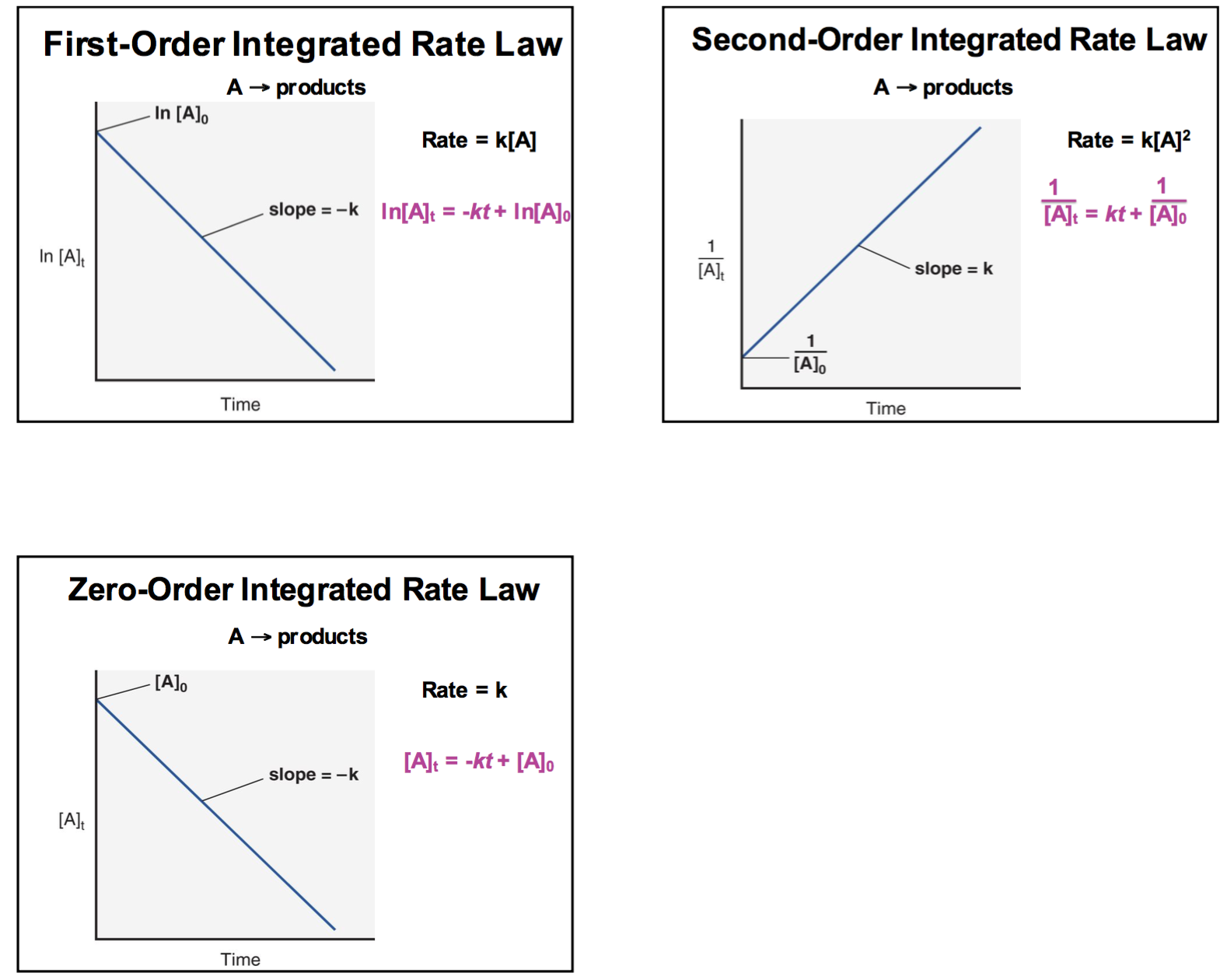## Graphs

Harmony Becerra
Posts: 32
Joined: Sun Jan 21, 2018 3:00 am

### Graphs

Just to clarify, the graph for a first order ln[X] vs time and zero order [X] vs time reaction is a decreasing linear slope. For second order it is an increasing linear slope. Right? So when would they be the increasing and decreasing exponential graphs?

Eva Guillory 2E
Posts: 44
Joined: Fri Sep 28, 2018 12:22 am

### Re: Graphs

Here is a diagram that you might find helpful:eden tefera 2B
Posts: 39
Joined: Fri Sep 28, 2018 12:21 am

### Re: Graphs

I think that you should always get a linear plot for 0,1,and 2 order reactions!

g orloff 1J
Posts: 45
Joined: Fri Sep 28, 2018 12:16 am

### Re: Graphs

you should always have a linear graph based on the order of the reaction.

josephperez_2C
Posts: 70
Joined: Wed Nov 15, 2017 3:04 am

### Re: Graphs

first, second, and zero order reactions have linear plots

Sydney Tay 2B
Posts: 64
Joined: Fri Sep 28, 2018 12:20 am

### Re: Graphs

All of the graphs for the ordered reactions should have linear plots. It just depends on what is on the axis that determines what is the order of the reaction.

Jasmine Chow 1F
Posts: 60
Joined: Fri Sep 28, 2018 12:16 am
Been upvoted: 1 time

### Re: Graphs

All the graphs will be linear however, the K sign for the slope will be different. Zero and first order graphs will have a negative slope while a second order graph will have a positive K slope.

juliasloan_4g
Posts: 67
Joined: Fri Sep 28, 2018 12:28 am

### Re: Graphs

all graphs are linear but the slopes and axis vary

Rogelio Bazan 1D
Posts: 64
Joined: Tue Nov 14, 2017 3:01 am

### Re: Graphs

To my understanding and looking at the textbook first order reactions will have a negative (-) slope, second-order reactions will have a positive (+) slope, and zero order reactions will have a negative (-) slope. This is due to the value of k either being positive or negative which affect the slope of the graph. Hope this helps.